# Dijkstra.ppt

7 Sep 2022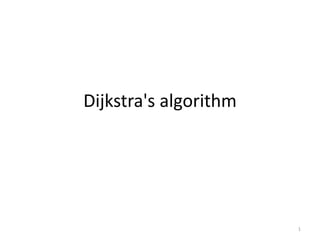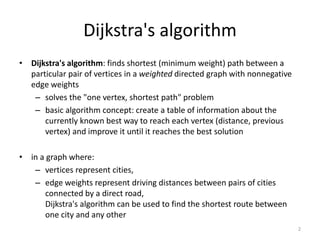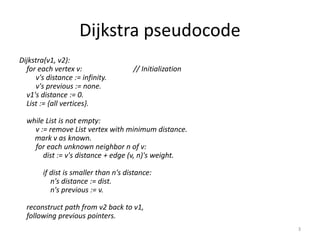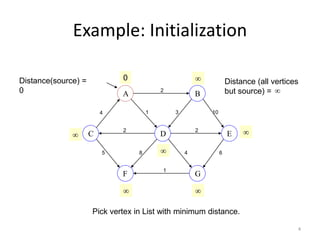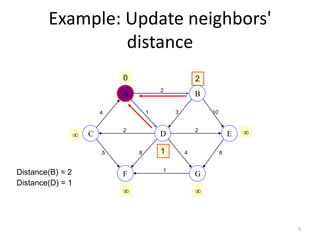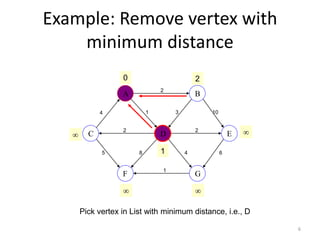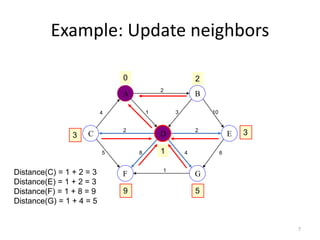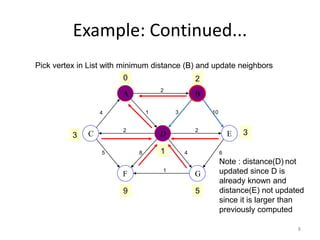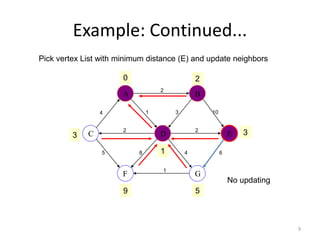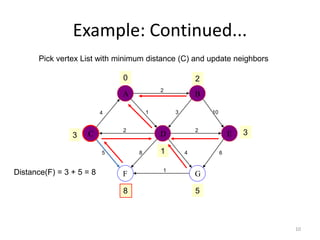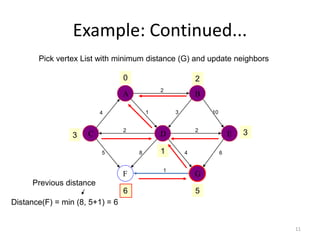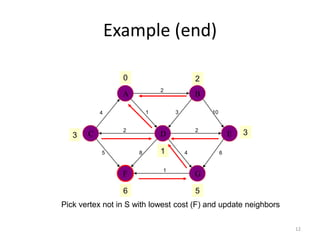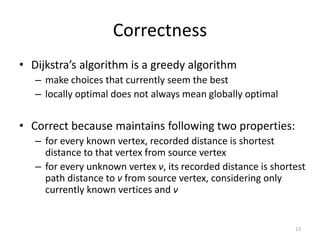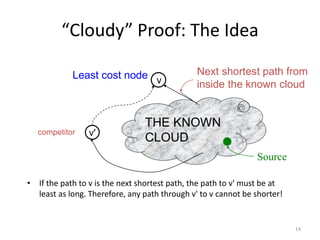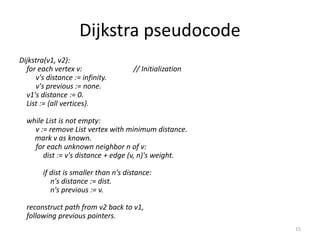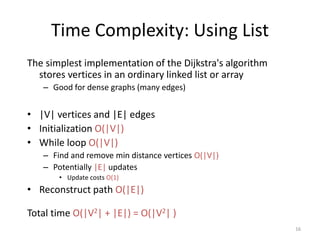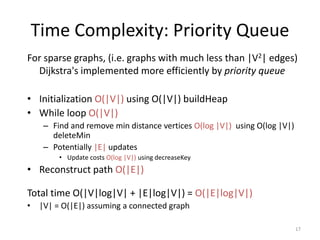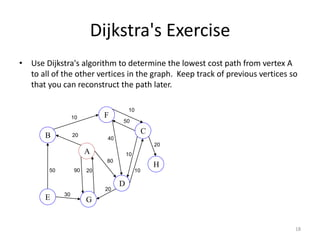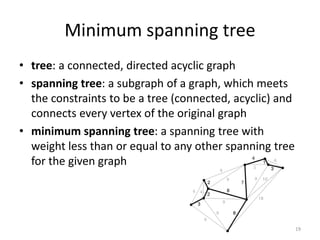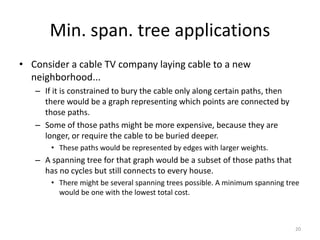1 sur 20

### Dijkstra.ppt

• 2. Dijkstra's algorithm • Dijkstra's algorithm: finds shortest (minimum weight) path between a particular pair of vertices in a weighted directed graph with nonnegative edge weights – solves the "one vertex, shortest path" problem – basic algorithm concept: create a table of information about the currently known best way to reach each vertex (distance, previous vertex) and improve it until it reaches the best solution • in a graph where: – vertices represent cities, – edge weights represent driving distances between pairs of cities connected by a direct road, Dijkstra's algorithm can be used to find the shortest route between one city and any other 2
• 3. Dijkstra pseudocode Dijkstra(v1, v2): for each vertex v: // Initialization v's distance := infinity. v's previous := none. v1's distance := 0. List := {all vertices}. while List is not empty: v := remove List vertex with minimum distance. mark v as known. for each unknown neighbor n of v: dist := v's distance + edge (v, n)'s weight. if dist is smaller than n's distance: n's distance := dist. n's previous := v. reconstruct path from v2 back to v1, following previous pointers. 3
• 4. 4 Example: Initialization A G F B E C D 4 1 2 10 3 6 4 2 2 8 5 1 0     Pick vertex in List with minimum distance.   Distance(source) = 0 Distance (all vertices but source) = 
• 5. 5 Example: Update neighbors' distance A G F B E C D 4 1 2 10 3 6 4 2 2 8 5 1 0 2   1   Distance(B) = 2 Distance(D) = 1
• 6. 6 Example: Remove vertex with minimum distance Pick vertex in List with minimum distance, i.e., D A G F B E C D 4 1 2 10 3 6 4 2 2 8 5 1 0 2   1  
• 7. 7 Example: Update neighbors A G F B E C D 4 1 2 10 3 6 4 2 2 8 5 1 0 2 3 3 1 9 5 Distance(C) = 1 + 2 = 3 Distance(E) = 1 + 2 = 3 Distance(F) = 1 + 8 = 9 Distance(G) = 1 + 4 = 5
• 8. 8 Example: Continued... A G F B E C D 4 1 2 10 3 6 4 2 2 8 5 1 0 2 3 3 1 Pick vertex in List with minimum distance (B) and update neighbors 9 5 Note : distance(D) not updated since D is already known and distance(E) not updated since it is larger than previously computed
• 9. 9 Example: Continued... A G F B E C D 4 1 2 10 3 6 4 2 2 8 5 1 0 2 3 3 1 9 5 No updating Pick vertex List with minimum distance (E) and update neighbors
• 10. 10 Example: Continued... A G F B E C D 4 1 2 10 3 6 4 2 2 8 5 1 0 2 3 3 1 8 5 Pick vertex List with minimum distance (C) and update neighbors Distance(F) = 3 + 5 = 8
• 11. 11 Example: Continued... A G F B E C D 4 1 2 10 3 6 4 2 2 8 5 1 0 2 3 3 1 6 5 Distance(F) = min (8, 5+1) = 6 Previous distance Pick vertex List with minimum distance (G) and update neighbors
• 12. 12 Example (end) A G F B E C D 4 1 2 10 3 6 4 2 2 8 5 1 0 2 3 3 1 Pick vertex not in S with lowest cost (F) and update neighbors 6 5
• 13. 13 Correctness • Dijkstra’s algorithm is a greedy algorithm – make choices that currently seem the best – locally optimal does not always mean globally optimal • Correct because maintains following two properties: – for every known vertex, recorded distance is shortest distance to that vertex from source vertex – for every unknown vertex v, its recorded distance is shortest path distance to v from source vertex, considering only currently known vertices and v
• 14. 14 THE KNOWN CLOUD v Next shortest path from inside the known cloud v' “Cloudy” Proof: The Idea • If the path to v is the next shortest path, the path to v' must be at least as long. Therefore, any path through v' to v cannot be shorter! Source Least cost node competitor
• 15. Dijkstra pseudocode Dijkstra(v1, v2): for each vertex v: // Initialization v's distance := infinity. v's previous := none. v1's distance := 0. List := {all vertices}. while List is not empty: v := remove List vertex with minimum distance. mark v as known. for each unknown neighbor n of v: dist := v's distance + edge (v, n)'s weight. if dist is smaller than n's distance: n's distance := dist. n's previous := v. reconstruct path from v2 back to v1, following previous pointers. 15
• 16. 16 Time Complexity: Using List The simplest implementation of the Dijkstra's algorithm stores vertices in an ordinary linked list or array – Good for dense graphs (many edges) • |V| vertices and |E| edges • Initialization O(|V|) • While loop O(|V|) – Find and remove min distance vertices O(|V|) – Potentially |E| updates • Update costs O(1) • Reconstruct path O(|E|) Total time O(|V2| + |E|) = O(|V2| )
• 17. 17 Time Complexity: Priority Queue For sparse graphs, (i.e. graphs with much less than |V2| edges) Dijkstra's implemented more efficiently by priority queue • Initialization O(|V|) using O(|V|) buildHeap • While loop O(|V|) – Find and remove min distance vertices O(log |V|) using O(log |V|) deleteMin – Potentially |E| updates • Update costs O(log |V|) using decreaseKey • Reconstruct path O(|E|) Total time O(|V|log|V| + |E|log|V|) = O(|E|log|V|) • |V| = O(|E|) assuming a connected graph
• 18. 18 Dijkstra's Exercise A G F B E C D 20 10 50 40 20 80 50 20 30 20 90 H 10 10 10 • Use Dijkstra's algorithm to determine the lowest cost path from vertex A to all of the other vertices in the graph. Keep track of previous vertices so that you can reconstruct the path later.
• 19. Minimum spanning tree • tree: a connected, directed acyclic graph • spanning tree: a subgraph of a graph, which meets the constraints to be a tree (connected, acyclic) and connects every vertex of the original graph • minimum spanning tree: a spanning tree with weight less than or equal to any other spanning tree for the given graph 19
• 20. Min. span. tree applications • Consider a cable TV company laying cable to a new neighborhood... – If it is constrained to bury the cable only along certain paths, then there would be a graph representing which points are connected by those paths. – Some of those paths might be more expensive, because they are longer, or require the cable to be buried deeper. • These paths would be represented by edges with larger weights. – A spanning tree for that graph would be a subset of those paths that has no cycles but still connects to every house. • There might be several spanning trees possible. A minimum spanning tree would be one with the lowest total cost. 20